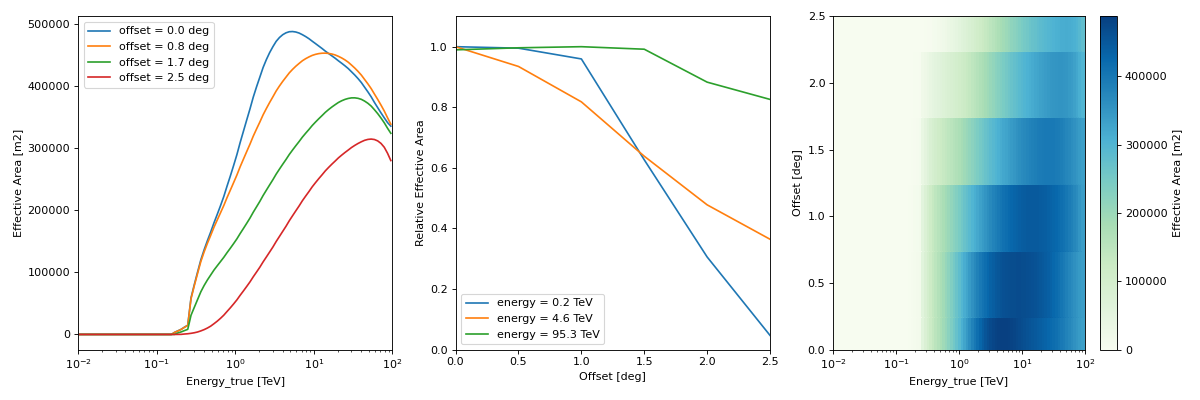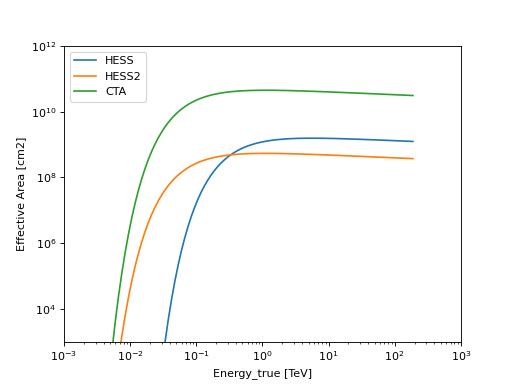# Effective area¶

## as a function of true energy and offset angle (AEFF_2D)¶

The EffectiveAreaTable2D class represents an effective area as a function of true energy and offset angle from the field of view center ($$A_{\rm eff}(E_{\rm true}, p_{\rm true})$$, following the notation in IRF Theory).

Its format specifications are available in AEFF_2D.

This is the format in which IACT DL3 effective areas are usually provided, as an example

"""Plot an effective area from the HESS DL3 data release 1."""
import matplotlib.pyplot as plt
from gammapy.irf import EffectiveAreaTable2D

filename = "\$GAMMAPY_DATA/hess-dl3-dr1/data/hess_dl3_dr1_obs_id_020136.fits.gz"
aeff.peek()
plt.show()


(png, hires.png, pdf)• using a pre-defined effective area parameterisation

from astropy import units as u
import matplotlib.pyplot as plt
from gammapy.irf import EffectiveAreaTable2D

for instrument in ["HESS", "HESS2", "CTA"]:
aeff = EffectiveAreaTable2D.from_parametrization(instrument=instrument)
ax = aeff.plot_energy_dependence(label=instrument, offset= * u.deg)

ax.set_yscale("log")
ax.set_xlim([1e-3, 1e3])
ax.set_ylim([1e3, 1e12])
plt.legend(loc="best")
plt.show()


(png, hires.png, pdf)